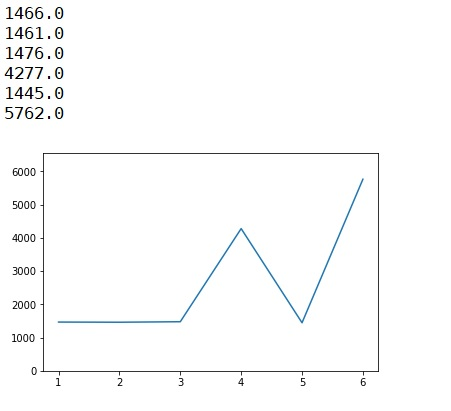# Python program for single biased dice simulation

Single biased dice simulation in Python: Here, we are going to learn how to simulate the occurrence of each dice face i.e. 1, 2, 3, 4, 4, 4, 5, 6, 6, 6, 6?
Submitted by Anuj Singh, on July 31, 2019

Here, we will be stimulating the occurrence of each dice face i.e. 1, 2, 3, 4, 4, 4, 5, 6, 6, 6, 6. Simply we are going to use an inbuilt library called as random to call a random value from given set and thereby we can stimulate the occurrence value by storing the occurrence in the list ls of length 6 representing each face of the dice as ls represents the occurrence of face 5.

```    ls - dice(1)
ls - dice(2)
ls - dice(3)
ls - dice(4)
ls - dice(5)
ls - dice(6)
```

Then using the library pylab, we can plot the value of each occurrence with respect to numbers of the hit. The probability of the dice faces is given below:

```    -    For face 1:
-    For face 2:
-    For face 3:
-    For face 4:
-    For face 5:
-    For face 6:
```

So the simulation of the occurrence of the dice over the number of times results in:

Program:

```import random
import pylab as py

def roll():
return random.choice([1,2,3,4,4,4,5,6,6,6,6])

ls = [0,0,0,0,0,0]
chance = [104, 203, 302, 401, 505, 646, 756, 855, 985, 4565, 6565]
for n in chance:
for k in range(n):
scr = roll()
ls[scr-1] = ls[scr-1] + 4/4

py.figure()
py.plot([1,2,3,4,5,6], ls)
py.ylim(0,6540)

for el in ls:
print(el)
```

OutputPreparation